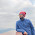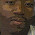.net & SQL Samples, programming tips and tricks, performance tips, guidelines, and best practices

## Wednesday, 17 October 2012

### SQL Server # Database Backup Report using T-SQL

Today, I am going to share few very useful scripts which will report us on Database Backup from different view points.

To get the List/History/Log of all the Successful Backups

`SELECT `
`  b.machine_name,`
`  b.server_name,`
`  b.database_name as DBName,`
`  b.backup_start_date,`
`  b.backup_finish_date,`
`  CASE `
`    WHEN b.[type] = 'D' THEN 'Database'`
`    WHEN b.[type] = 'I' THEN 'Differential database'`
`    WHEN b.[type] = 'L' THEN 'Log'`
`    WHEN b.[type] = 'F' THEN 'File or filegroup'`
`    WHEN b.[type] = 'G' THEN 'Differential file'`
`    WHEN b.[type] = 'P' THEN 'Partial'`
`    WHEN b.[type] = 'Q' THEN 'Differential partial'`
`    ELSE b.[type]`
`  END Backup_Type,    `
`  b.expiration_date,`
`  b.[user_name],`
`  DATEDIFF(MINUTE,b.backup_start_date ,b.backup_finish_date) as Total_Time_in_Minute,`
`  b.recovery_model,`
`  b.backup_size/(1024 * 1024 * 1024) as Total_Size_GB,`
`  bf.physical_device_name as Location`
`FROM `
`  msdb.dbo.backupset AS b`
`INNER JOIN msdb.dbo.backupmediafamily AS bf`
`  ON b.media_set_id=bf.media_set_id`
`ORDER BY `
`  b.backup_start_date DESC`
`GO`

To get a list of all successful Backups taken till date for a particular Database

`DECLARE @DBName AS VARCHAR(100) = 'Your Database Name'`
` `
`SELECT `
`  b.machine_name,`
`  b.server_name,`
`  b.database_name as DBName,`
`  b.backup_start_date,`
`  b.backup_finish_date,`
`  CASE `
`    WHEN b.[type] = 'D' THEN 'Database'`
`    WHEN b.[type] = 'I' THEN 'Differential database'`
`    WHEN b.[type] = 'L' THEN 'Log'`
`    WHEN b.[type] = 'F' THEN 'File or filegroup'`
`    WHEN b.[type] = 'G' THEN 'Differential file'`
`    WHEN b.[type] = 'P' THEN 'Partial'`
`    WHEN b.[type] = 'Q' THEN 'Differential partial'`
`    ELSE b.[type]`
`  END Backup_Type,`
`  b.expiration_date,`
`  b.[user_name],`
`  DATEDIFF(MINUTE,b.backup_start_date ,b.backup_finish_date) as Total_Time_in_Minute,`
`  b.recovery_model,`
`  b.backup_size/(1024 * 1024 * 1024) as Total_Size_GB,`
`  bf.physical_device_name as Location`
`FROM `
`  msdb.dbo.backupset AS b`
`INNER JOIN msdb.dbo.backupmediafamily AS bf`
`  ON b.media_set_id=bf.media_set_id`
`WHERE`
`  b.database_name = @DBName  `
`ORDER BY `
`  b.backup_start_date DESC`
`GO`

To get the List of all Databases which are not backed up till date

`SELECT`
`  d.name [DB_Name]`
`FROM`
`  master.sys.databases d`
`LEFT JOIN msdb.dbo.backupset b`
`  ON b.database_name = d.name`
`WHERE`
`  d.database_id IS NULL`

To get the List of all Databases which are not backed up since last X days

`DECLARE @LastXDays AS INT = 1`
`;WITH LatestBackupSet AS (`
`SELECT `
`  b.database_name as DBName,`
`  b.backup_start_date LastBackedUpOn,`
`  b.[user_name],`
`  ROW_NUMBER() OVER(PARTITION BY b.database_name ORDER BY b.backup_start_date DESC) Rnk`
`FROM `
`  msdb.dbo.backupset AS b`
`)`
`SELECT `
`  lbs.DBName,`
`  lbs.LastBackedUpOn,`
`  lbs.[user_name]`
`FROM `
`  LatestBackupSet AS lbs`
`WHERE`
`  DATEDIFF(DAY,lbs.LastBackedUpOn ,CURRENT_TIMESTAMP) = @LastXDays  `
`  AND lbs.Rnk = 1`
`ORDER BY `
`  lbs.DBName DESC`
`GO`

To get a list of the Latest successful backups of all Databases

`;WITH LatestBackupSet AS (`
`SELECT `
`  b.machine_name,`
`  b.server_name,`
`  b.database_name as DBName,`
`  b.backup_start_date,`
`  b.backup_finish_date,`
`  CASE `
`    WHEN b.[type] = 'D' THEN 'Database'`
`    WHEN b.[type] = 'I' THEN 'Differential database'`
`    WHEN b.[type] = 'L' THEN 'Log'`
`    WHEN b.[type] = 'F' THEN 'File or filegroup'`
`    WHEN b.[type] = 'G' THEN 'Differential file'`
`    WHEN b.[type] = 'P' THEN 'Partial'`
`    WHEN b.[type] = 'Q' THEN 'Differential partial'`
`    ELSE b.[type]`
`  END Backup_Type,`
`  b.expiration_date,`
`  b.[user_name],`
`  DATEDIFF(MINUTE,b.backup_start_date ,b.backup_finish_date) as Total_Time_in_Minute,`
`  b.recovery_model,`
`  b.backup_size/(1024 * 1024 * 1024) as Total_Size_GB,`
`  bf.physical_device_name as Location,`
`  ROW_NUMBER() OVER(PARTITION BY b.database_name ORDER BY b.backup_start_date DESC) Rnk`
`FROM `
`  msdb.dbo.backupset AS b`
`INNER JOIN msdb.dbo.backupmediafamily AS bf`
`  ON b.media_set_id=bf.media_set_id`
`)`
`SELECT `
`  machine_name,`
`  server_name,`
`  DBName,`
`  backup_start_date,`
`  backup_finish_date,`
`  Backup_Type,`
`  expiration_date,`
`  [user_name],`
`  Total_Time_in_Minute,`
`  recovery_model,`
`  Total_Size_GB,`
`  Location`
`FROM `
`  LatestBackupSet AS lbs`
`WHERE`
`  lbs.Rnk = 1`
`ORDER BY `
`  lbs.DBName DESC`
`GO`

To get the Latest successful backup of a particular Database

`DECLARE @DBName AS VARCHAR(100) = 'Your Database Name'`
` `
`;WITH LatestBackupSet AS (`
`SELECT `
`  b.machine_name,`
`  b.server_name,`
`  b.database_name as DBName,`
`  b.backup_start_date,`
`  b.backup_finish_date,`
`  CASE `
`    WHEN b.[type] = 'D' THEN 'Database'`
`    WHEN b.[type] = 'I' THEN 'Differential database'`
`    WHEN b.[type] = 'L' THEN 'Log'`
`    WHEN b.[type] = 'F' THEN 'File or filegroup'`
`    WHEN b.[type] = 'G' THEN 'Differential file'`
`    WHEN b.[type] = 'P' THEN 'Partial'`
`    WHEN b.[type] = 'Q' THEN 'Differential partial'`
`    ELSE b.[type]`
`  END Backup_Type,`
`  b.expiration_date,`
`  b.[user_name],`
`  DATEDIFF(MINUTE,b.backup_start_date ,b.backup_finish_date) as Total_Time_in_Minute,`
`  b.recovery_model,`
`  b.backup_size/(1024 * 1024 * 1024) as Total_Size_GB,`
`  bf.physical_device_name as Location,`
`  ROW_NUMBER() OVER(PARTITION BY b.database_name ORDER BY b.backup_start_date DESC) Rnk`
`FROM `
`  msdb.dbo.backupset AS b`
`INNER JOIN msdb.dbo.backupmediafamily AS bf`
`  ON b.media_set_id=bf.media_set_id`
`WHERE`
`  b.database_name = @DBName  `
`)`
`SELECT `
`  machine_name,`
`  server_name,`
`  DBName,`
`  backup_start_date,`
`  backup_finish_date,`
`  Backup_Type,`
`  expiration_date,`
`  [user_name],`
`  Total_Time_in_Minute,`
`  recovery_model,`
`  Total_Size_GB,`
`  Location`
`FROM `
`  LatestBackupSet AS lbs`
`WHERE`
`  lbs.Rnk = 1`
`ORDER BY `
`  lbs.DBName DESC`
`GO`

To get a list of Databases that were backed-up and do not currently exist

`SELECT`
`  DISTINCT b.database_name`
`FROM`
`  msdb.dbo.backupset b`
`WHERE`
`  DB_ID(b.database_name) IS NULL`

Hope, the above given script will be of help to you. Also, I would like to request you to please add any relevant script which you feel would be useful as a comment.

1.Very Useful :)

2.Hi Vinay

I need to extract some data but I am failing because Ii don't know what I need to add on the sql instance. How can I knwo my sql instance so that I add it to the procedure?

Kind Regards,
Emmanuels

3.Hello Emmanuel,
Can you please elaborate more on your problem statement. I am not very clear on where you are stuck.